Next: Darwin-Radau equation Up: Yielding of an elastic Previous: Gravitational potential theory

# Elastic response theory

The interior of the planet is modeled as a uniform, incompressible, elastic solid possessing the isotropic stress-strain relation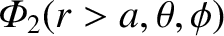(C.21)

and subject to the incompressibility constraint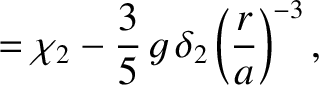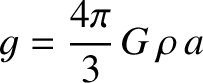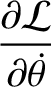(C.22)

Here,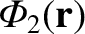is the stress tensor,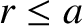the identity tensor,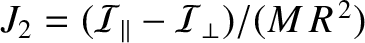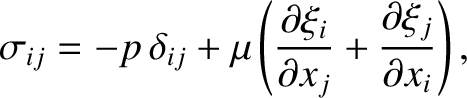the elastic displacement,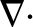the pressure, and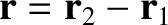the (uniform) rigidity of the material making up the planet (Riley 1974e).

Force balance inside the planet yields (Love 2011)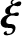(C.23)

It follows from Equations (C.21) and (C.22) that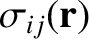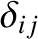(C.24)

Writing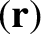(C.25)

Equation (C.24) yields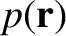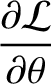(C.26) and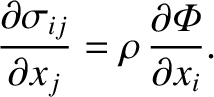(C.27)

Taking the divergence of the previous equation, and making use of Equation (C.22), we find that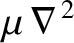, which implies that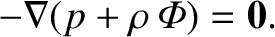is a solid harmonic (of degree 2). Incidentally,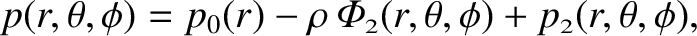would be zero were the planet in hydrostatic equilibrium.

It is helpful to define the radial component of the elastic displacement,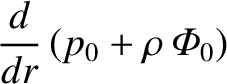(C.28)

as well as the stress acting (outward) across a constant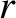surface,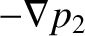(C.29)

where use has been made of Equation (C.21). Of course, the radial displacement at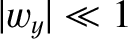is equivalent to the displacement of the planet's surface: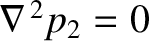(C.30)

The stress at any point on the surfacemust be entirely radial (because it would be impossible to balance a tangential surface stress), and such as to balance the weight of the column of displaced material directly above the point in question. In other words,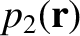(C.31)

It follows from Equations (C.18), (C.25), and (C.29) that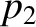(C.32) and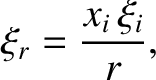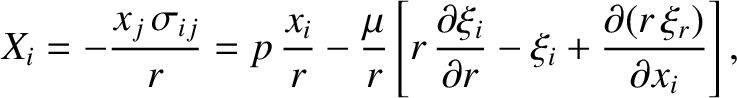(C.33)

where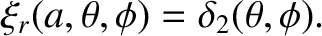(C.34)

Equations (C.16), (C.26), and (C.32) yield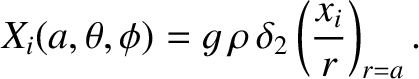(C.35)

It remains to solve Equations (C.22) and (C.27), subject to the boundary conditions (C.30) and (C.33).

Let us try a solution to Equations (C.22) and (C.27) of the form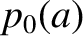(C.36)

where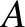and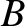are spatial constants, and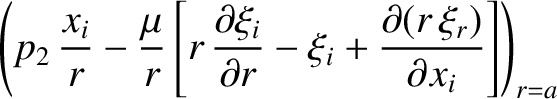is a solid harmonic of degree 2 (Love 2011). It follows that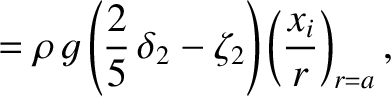(C.37)

where use has been made of Equation (C.5). Moreover,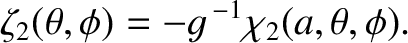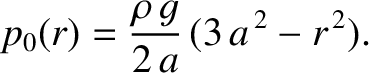(C.38) and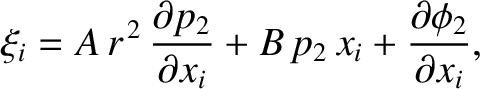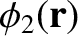(C.39)

where use has been made of Equations (C.5)-(C.7). Thus, the boundary conditions (C.30) and (C.33) become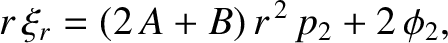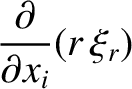(C.40) and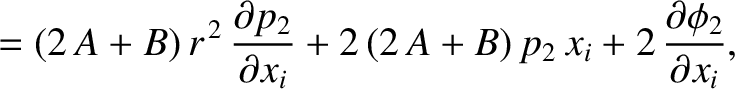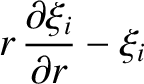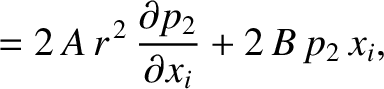(C.41)

respectively. The previous equation implies that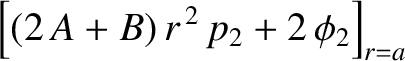(C.42)

Hence, the boundary conditions (C.40) and (C.41) reduce to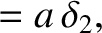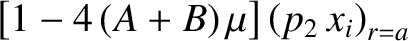(C.43) and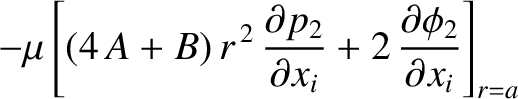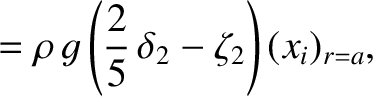(C.44)

respectively.

The expression for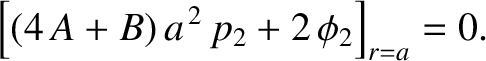given in Equation (C.36) satisfies Equations (C.22) and (C.27) provided that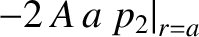(C.45) and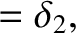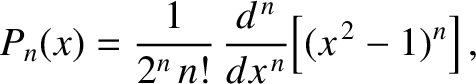(C.46)

respectively, where use has been made of Equations (C.5)-(C.7). It follows that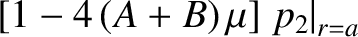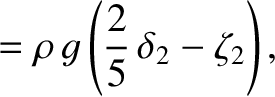(C.47) and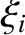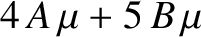(C.48)

Hence, the boundary conditions (C.43) and (C.44) yield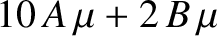(C.49)

where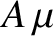(C.50)

The dimensionless quantity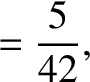is termed a Love number of degree 2 (Love 2011).

The radial component of the elastic (i.e., non-hydrostatic) stress acting (outward) across the surfacetakes the form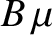(C.51)

where use has been made of Equation (C.33). Equations (C.49) and (C.50) imply that this stress is related to the radial strain at the surface of the planet according to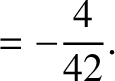(C.52)

As a specific example, suppose that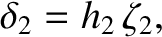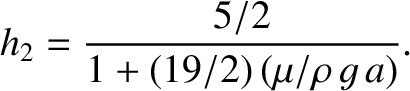(C.53)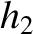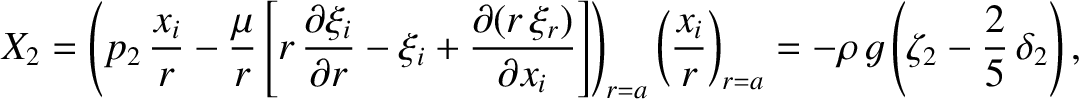(C.54) and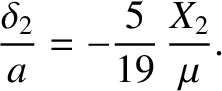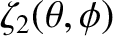(C.55)

where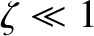is a dimensionless measure of the strength of the tidal field, and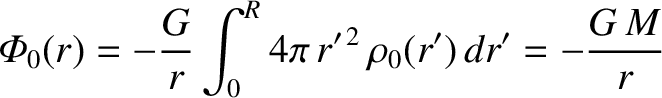is the tidally induced planetary ellipticity. It follows that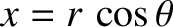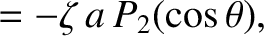(C.56) and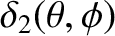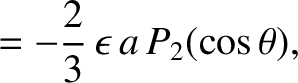(C.57)

where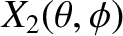(C.58)

is the planet's effective rigidity.Next: Darwin-Radau equation Up: Yielding of an elastic Previous: Gravitational potential theory
Richard Fitzpatrick 2016-03-31